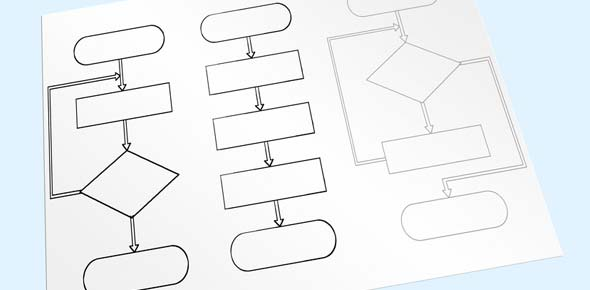# Dsa_1

10 Questions | Total Attempts: 39SettingsRelated Topics
• 1.
Among Breadth-first search and Depth-first search, which graph traversal algorithm uses a queue to keep track of vertices that need to be processed?
• A.

Both Breadth-first search and Depth-first search

• B.

None of them

• C.

Only Depth-First Search

• D.

• 2.
A list of four numbers (4, 1, 3, 2) was re-ordered in ascending order in accordance with a sorting algorithm. The numbers were re-ordered as shown below. Which of the following is the sorting algorithm? (1, 4, 3, 2) (1, 3, 4, 2) (1, 2, 3, 4)
• A.

Selection sort

• B.

Insertion sort

• C.

Bubble sort

• D.

Quick sort

• 3.
Which of the following represents the sequence of nodes visited in a post-order traversal of the binary tree T shown below?
• A.

U X Z Q W Y V P

• B.

U X W Q Z Y V P

• C.

U Q X W P V Z Y

• D.

X Z U W Y Q V P

• 4.
What does a run-time analysis usually count?
• A.

The number of seconds plus the number of megabytes

• B.

The number of arithmetic and other operations required for the program to run

• C.

The number of seconds required for the program to run

• D.

The number of megabytes required for the program to run

• 5.
Define a recursive function F( n ) as following: If n > 0 then F( n ) = n x F(n-1) If n = 0 then F( n ) = 1. As such, what is the value of F(5)?
• A.

5

• B.

120

• C.

1

• D.

15

• 6.
There are two important operations on a stack: PUSH and POP. PUSH adds the new data to the top of the stack leaving previous data below, and POP removes and returns the current top data of the stack. When the operations shown below are sequentially executed, which of the following is the correct combination of the values x and y? Here, the size of the stack is big enough to hold the entire data. “PUSH(a)” inserts the data a into the stack, and “POP(b)” removes the data b from the stack. [Operations] PUSH (5); PUSH (3); PUSH (6); PUSH (1); x = POP (  ); PUSH (7); y = POP (  ); What are the value of X and Y?
• A.

• B.

• C.

• D.

• 7.
Which of the following stack operations could result in stack underflow?
• A.

Top

• B.

Pop

• C.

Push

• D.

Is_empty

• 8.
Given a tree T in the box. What is the order of nodes visited using a pre-order traversal?
• A.

R S X Y Z T U W P V

• B.

R S T X Y U V Z W P

• C.

X S Z Y R W P U T V

• D.

X Z Y S P W U V T R

• 9.
Given a graph G in the box. What is the order of nodes visited using DFS, starting from node a
• A.

A f e d c k g b h i j l

• B.

A c d e f k b g h i j l

• C.

A b c d e f k g h i j l

• D.

A c b g l k h i j f e d

• 10.
Suppose cursor points to a node in a linked list (using the node definition with member functions called data and link). What Boolean expression will be true when cursor points to the tail node of the list?
• A.